# Properties of Relations

Assumptions are the termites of relationships.

character of Arthur Fonzarelli, Happy Days

Now we are ready to consider some properties of relations. Our interest is to find properties of, e.g. motherhood. To do this, remember that we are not interested in a particular mother or a particular child, or even in a particular mother-child pair, but rather motherhood in general.

We'll start with properties that make sense for relations whose source and target are the same, that is, relations on a set.

Definition. Let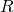be a relation on the set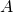.

• We callreflexive if every element ofis related to itself; that is, if every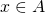has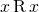.
• We callirreflexive if no element ofis related to itself.
• We callsymmetric if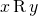means the same thing as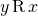.
• We callasymmetric ifguarantees that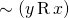.
• We callantisymmetric if the only way forandto both be true is if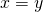.
• We calltransitive ifandtogether guarantee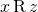.

Exercise. Explain why none of these relations makes sense unless the source and target ofare the same set.

Exercise. Which of the above properties does the motherhood relation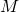have?

Exercise. Write the definitions above using set notation instead of infix notation.

Exercise. Write the definitions of reflexive, symmetric, and transitive using logical symbols.

Checking whether a given relation has the properties above looks like:

E.g. Divides' (as a relation on the integers) is reflexive and transitive, but none of: symmetric, asymmetric, antisymmetric.

Proof. We'll show reflexivity first. Suppose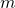is an integer. Then, sodivides.

Now we'll show transitivity. Supposedividesanddivides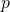. Then there are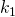and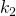so that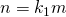and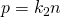. Then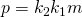, sodivides.

Note that 2 divides 4 but 4 does not divide 2. This counterexample shows that divides' is not symmetric.

Note that 4 divides 4. This counterexample shows that divides' is not asymmetric.

Note that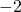divides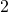anddivides, but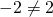. This counterexample shows that divides' is not antisymmetric.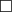E.g.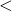is irreflexive, asymmetric, transitive, and antisymmetric, but neither reflexive nor symmetric.

Exercise. Show that `divides' as a relation on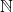is antisymmetric.